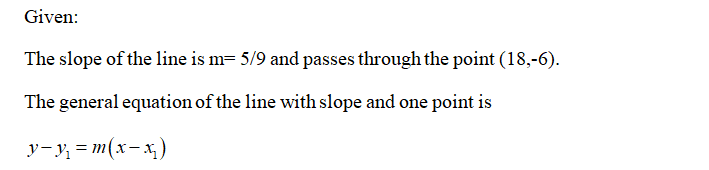# Write the equation of the line that has the slope m= 5/9 and passes through the point (18,-6). Write the equation in​ slope-intercept form if possible.

Question
1 views

Write the equation of the line that has the slope m= 5/9 and passes through the point (18,-6). Write the equation in​ slope-intercept form if possible.

check_circle

Step 1...

### Want to see the full answer?

See Solution

#### Want to see this answer and more?

Solutions are written by subject experts who are available 24/7. Questions are typically answered within 1 hour.*

See Solution
*Response times may vary by subject and question.
Tagged in

### Algebra